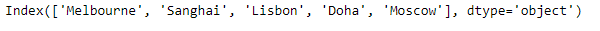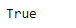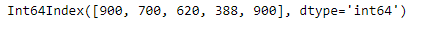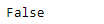GFG App
Open AppBrowser
Continue

# Python | Pandas Index.is_unique

Pandas Index is an immutable ndarray implementing an ordered, sliceable set. It is the basic object which stores the axis labels for all pandas objects.

Pandas` Index.is_unique` attribute return `True` if the underlying data in the given Index object is unique else it return `False`.

Syntax: Index.is_unique

Parameter : None

Returns : boolean

Example #1: Use `Index.is_unique` attribute to find out if the underlying data in the given Index object is unique or not.

 `# importing pandas as pd ` `import` `pandas as pd ` ` `  `# Creating the index ` `idx ``=` `pd.Index([``'Melbourne'``, ``'Sanghai'``, ``'Lisbon'``, ``'Doha'``, ``'Moscow'``]) ` ` `  `# Print the index ` `print``(idx) `

Output :Now we will use `Index.is_unique` attribute to find out if the underlying data in the given Index object is unique or not.

 `# check if the values in the Index ` `# is unique or not. ` `result ``=` `idx.is_unique ` ` `  `# Print the result ` `print``(result) `

Output :As we can see in the output, the `Index.is_unique` attribute has returned `True` indicating that the underlying data of the given Index object is unique.

Example #2 : Use `Index.is_unique` attribute to find out if the underlying data in the given Index object is unique or not.

 `# importing pandas as pd ` `import` `pandas as pd ` ` `  `# Creating the index ` `idx ``=` `pd.Index([``900``, ``700``, ``620``, ``388``, ``900``]) ` ` `  `# Print the index ` `print``(idx) `

Output :Now we will use `Index.is_unique` attribute to find out if the underlying data in the given Index object is unique or not.

 `# check if the values in the Index ` `# is unique or not. ` `result ``=` `idx.is_unique ` ` `  `# Print the result ` `print``(result) `

Output :As we can see in the output, the `Index.is_unique` attribute has returned `False` indicating that the underlying data of the given Index object is not unique.

My Personal Notes arrow_drop_up